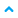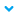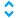# Engineering unit converter

Use the engineering unit converter to convert raw data values into scaled values based on a range of customizable values.
TIP: For example, the engineering unit converter can transform values from 0 to 65.535 supplied by a temperature sensor into corresponding values on a scale from -20 to +70. The input of an engineering unit converter is generally a dynamic link to an imported tag.

## Properties

Property
Description
Raw min
Minimum raw data value.
Raw max
Maximum raw data value.
Scaled min
Minimum converted value.
Scaled max
Maximum converted value.
Mode
The direction of the dynamic link:
•Read from the source node to the parent.
•Write from the parent to the source node.
•Read from the source node to the parent and write from the parent to the source node.
IMPORTANT: The converter does not operate if you do not specify values in the
Raw min
and
Raw max
properties and there is no dynamic link for the input variable.
If...
Then...
Raw min
and
Raw max
properties are not set.
The converter assumes that there is an analog variable set in the input via a dynamic link.
Raw min
and
Raw max
are set to
Low limit
and
High limit
of the input analog variable respectively.
Raw min
and
Raw max
properties are set.
The converter considers
Raw min
and
Raw max
properties and ignores any
Low limit
and
High limit
values of the analog variable input.
Only
Raw min
or
Raw max
property is set.
The converter assumes that there is an analog variable set in the input via a dynamic link and ignores the
Raw min
and
Raw max
properties.
Raw min
and
Raw max
are set to
Low limit
and
High limit
of the input analog variable respectively.

## Source

A variable that contains a raw value to be converted.

## Output

A scaled value based on the configured linear conversion.

Provide Feedback